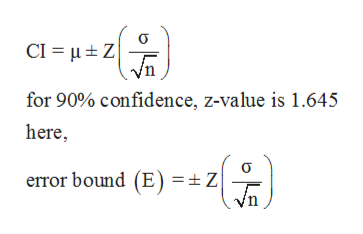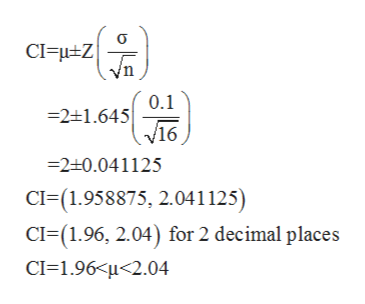# A sample of 16 small bags of the same brand of candies was selected. Assume that the population distribution of bag weights is normal. The weights of each bag was then recorded. The mean weight was two ounces with a standard deviation of 0.12 ounces. The population standard deviation is known to be 0.1 ounce.Construct a 90% confidence interval for the population mean weight of the candies.What is the error bound? (Round to three decimal places)

Question
1 views

A sample of 16 small bags of the same brand of candies was selected. Assume that the population distribution of bag weights is normal. The weights of each bag was then recorded. The mean weight was two ounces with a standard deviation of 0.12 ounces. The population standard deviation is known to be 0.1 ounce.

Construct a 90% confidence interval for the population mean weight of the candies.

What is the error bound? (Round to three decimal places)

check_circle

Step 1

Given information is below,

Sample size (n) = 16,

Mean (μ) = 2 ounces,

Population standard deviation (σ) = 0.1 ounce.

Step 2

Formula for confidence interval for population mean is,help_outlineImage TranscriptioncloseCI Z for 90% confidence, z-value is 1.645 here error bound (E) = Z fullscreen
Step 3

Now, substitute given values...help_outlineImage Transcriptionclose0.1 21.645 /16 2+0.041125 CI-(1.958875, 2.041125) CI (1.96, 2.04) for 2 decimal places CI 1.96 u<2.04 이들 fullscreen

### Want to see the full answer?

See Solution

#### Want to see this answer and more?

Solutions are written by subject experts who are available 24/7. Questions are typically answered within 1 hour.*

See Solution
*Response times may vary by subject and question.
Tagged in

### Statistics